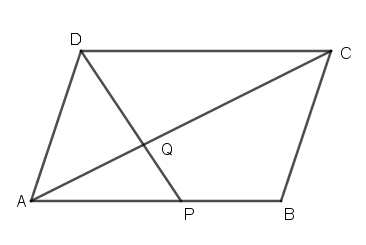QuestionAnswers

# The diagonal AC of a parallelogram ABCD intersects DP at the point Q, where P is any point on side AB. Prove that $CQ \times PQ = QA \times QD$.

Hint: In the above question we have a parallelogram in which their opposite sides are parallel with each other. We have a diagonal $AC$ which intersects $DP$ at the point $Q$, where $P$ is any point on side $AB$. In this question, we will use the concept of a similar triangle.

Complete step by step solution:
Given, $ABCD$ is a parallelogram.
$AC$ is a diagonal intersects $DP$ at $Q$ and $P$ is a point on side $AB$.
Now, we have drawn a figure, according to the given situation in question.To prove: $CQ \times PQ = QA \times QD$.
In $\vartriangle CQD$and $\vartriangle APQ$, we have;
$\angle CQD = \angle AQP$ (Vertically opposite angles and AB || CD)
$\angle QCD = \angle QAP$ (Alternate angles)
Thus, $\vartriangle CQD \sim \vartriangle APQ$(both the triangle is similar by angle-angle criterion)
$\Rightarrow \dfrac{{PQ}}{{QD}} = \dfrac{{QA}}{{CQ}}$
[If two triangles are similar, then the ratio of their corresponding sides are equal]
$\Rightarrow PQ \times CQ = QA \times QD$

$\therefore$Hence, it is proved that $CQ \times PQ = QA \times QD$.

Note: In this question we have used the concept of similar triangles. Two triangles are similar if their corresponding sides are in proportion and the corresponding angles are congruent. Similar triangles have the same shape but it is not necessary that they have the same size. In this question, we have used Angle-Angle Similarity in which if two angles of a triangle are equal to any angles of another triangle, then these two triangles are said to be similar triangles. There are some other criteria to prove Similarity like Side-Side-Side Similarity (SSS Similarity). There is an important theorem i.e., Thales theorem or Basic Proportionality Theorem in which two equiangular triangles, the ratio of any two corresponding sides is always the same.